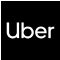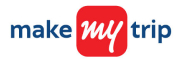New update is available. Click here to update.
Topics

# Number of Islands II

Hard0/120
Average time to solve is 45m+4 more companies

## Problem Statement

You have a 2D grid of ‘N’ rows and ‘M’ columns which are initially filled with water. You are given ‘Q’ queries each consisting of two integers ‘X’ and ‘Y’ and in each query operation, you have to turn the water at position (‘X’, ‘Y’) into a land. You are supposed to find the number of islands in the grid after each query.

An island is a group of lands surrounded by water horizontally, vertically, or diagonally.

Detailed explanation ( Input/output format, Notes, Images )
Constraints:
``````1 <= T <= 5
1 <= N <= 1000
1 <= M <= 1000
1 <= Q <= 100000
0 <= X < N
0 <= Y < M

Time limit: 1 sec
``````
Sample Input 1:
``````2
3 3
4
0 0
0 1
1 2
2 1
4 5
4
1 1
0 1
3 3
3 4
``````
Sample Output 1:
``````1 1 2 3
1 1 2 2
``````
Explanation of Sample Output 1:
``````In test case 1,

0.  000
000
000

1.  100
000
000

2.  110
000
000

3. 110
001
000

4. 110
001
100

So, the answer is 1, 1, 2, 3.

In test case 2,

0.  00000
00000
00000
00000

1.  00000
01000
00000
00000

2.  01000
01000
00000
00000

3. 01000
01000
00000
00010

4. 01000
01000
00000
00011

So, the answer is 1, 1, 2, 2.
``````
Sample Input 2:
``````2
2 2
2
0 0
1 1
1 1
1
0 0
``````
Sample Output 2:
``````1 2
1
``````
Explanation of Sample Output 2:
``````In test case 1,

0.  00
00

1.  10
00

2.  10
01

So, the answer is 1, 2.

In test case 2,

0.  0

1.  1